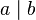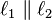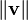# Vertical bar facts for kids

Kids Encyclopedia Facts

A vertical bar is the glyph "|". It has several other names besides vertical bar, such as "pipe", "vertical slash", and "bar". In mathematics, it may be put on both sides of a real or complex number to mean its absolute value. When applied on both sides of a matrix, it means its determinant. When placed in between two numbers, it means the divisibility relation (for example.$a \mid b$ means "a divides b").

In mathematics, double vertical bars are also used to refer to various mathematical concepts. These include the parallel relation (as in$\ell_1 \parallel \ell_2$) and the norm of a vector (as in$\| \mathbf{v}\|$).

## Images for kidsVertical bar Facts for Kids. Kiddle Encyclopedia.